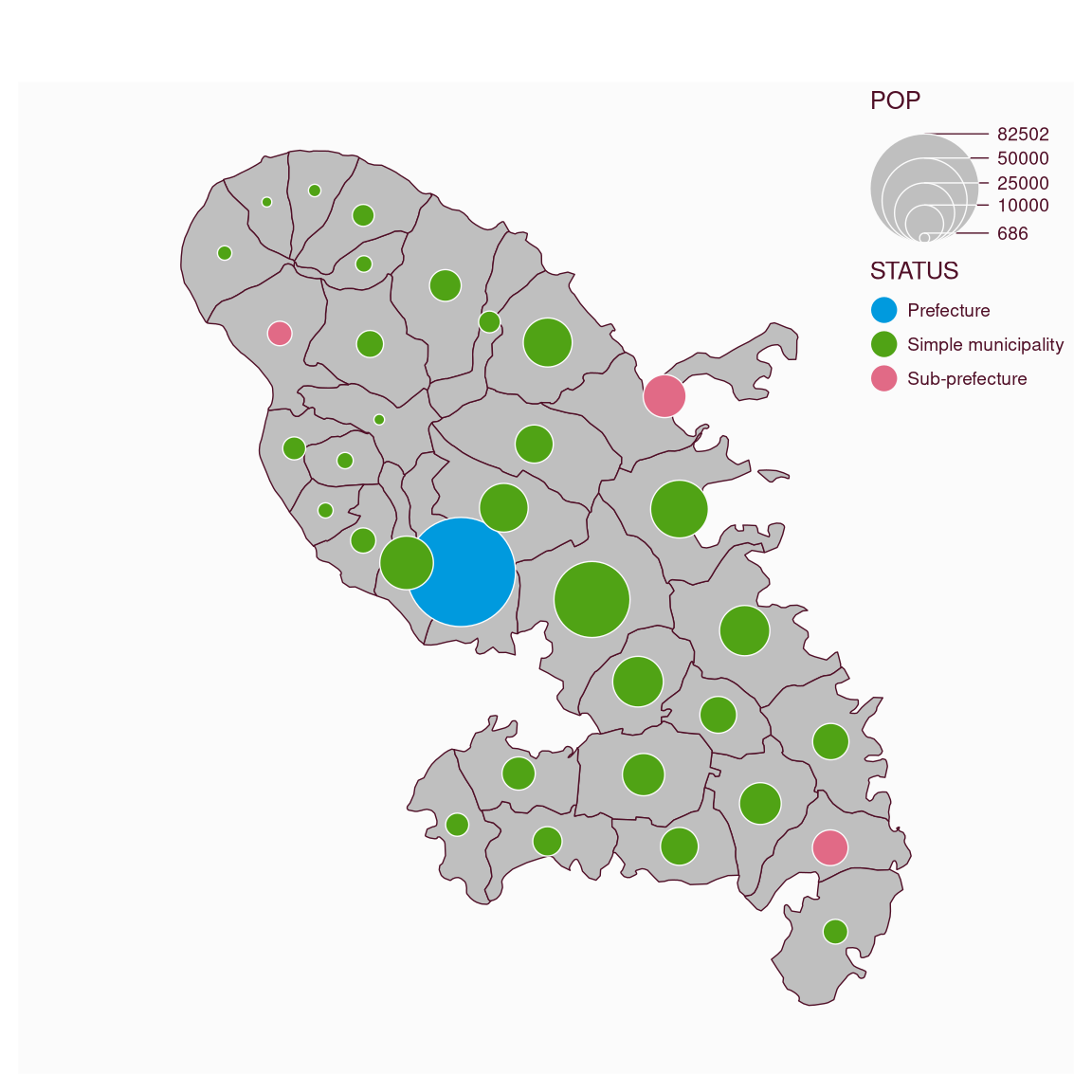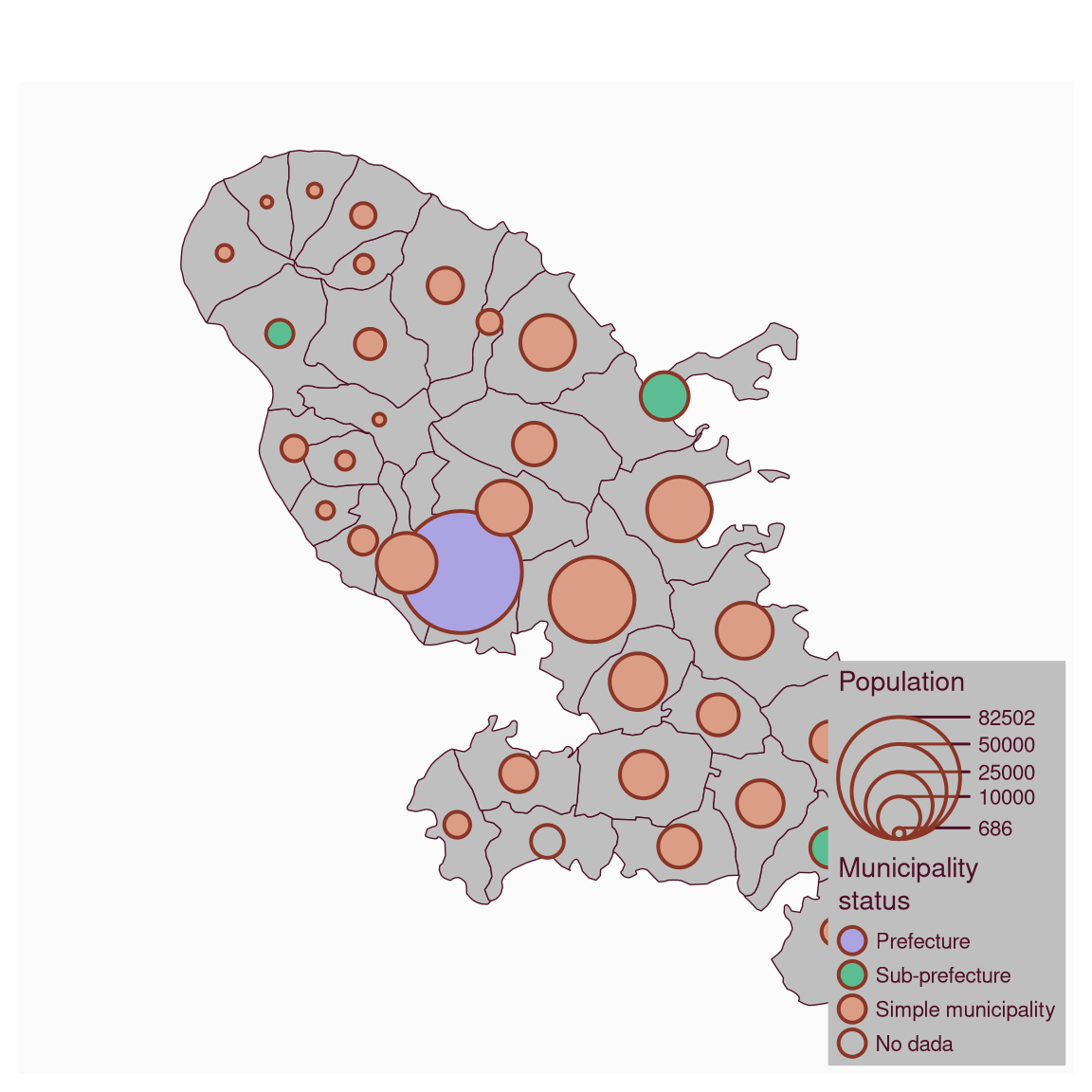Plot proportional symbols with colors based on qualitative data.

mf_prop_typo(
x,
var,
inches = 0.3,
val_max,
symbol = "circle",
pal = "Dynamic",
alpha = 1,
val_order,
border,
lwd = 0.7,
lwd_max = 15,
col_na = "white",
leg_pos = mf_get_leg_pos(x, 2),
leg_title = var,
leg_title_cex = c(0.8, 0.8),
leg_val_cex = c(0.6, 0.6),
leg_val_rnd = c(0),
leg_no_data = "No data",
leg_frame = c(FALSE, FALSE),
)

## Arguments

x

object of class sf

var

name(s) of the variable(s) to plot

inches

size of the biggest symbol (radius for circles, half width for squares) in inches.

val_max

maximum value used for proportional symbols

symbol

type of symbols, 'circle' or 'square'

pal

a set of colors or a palette name (from hcl.colors)

alpha

if pal is a hcl.colors palette name, the alpha-transparency level in the range [0,1]

val_order

values order, a character vector that matches var modalities

border

border color

lwd

border width

lwd_max

line width of the largest line

col_na

color for missing values

leg_pos

position of the legend, two of 'topleft', 'top','topright', 'right','bottomright', 'bottom', 'bottomleft', 'left' or a vector of two coordinates in map units (c(x, y)). leg_pos argument can be c('position', 'position'), c('position', x2, y2), c(x1,y1, 'position') or c(x1, y1, x2, y2). Use NA to avoid plotting the legend, use 'interactive' to choose thelegend position interactively.

leg_title

legend title

leg_title_cex

size of the legend title

leg_val_cex

size of the values in the legend

leg_val_rnd

number of decimal places of the values in the legend

leg_no_data

label for missing values

leg_frame

whether to add a frame to the legend (TRUE) or not (FALSE)

whether to add the layer to an existing plot (TRUE) or not (FALSE)

## Value

x is (invisibly) returned.

## Examples

mtq <- mf_get_mtq()
mf_map(mtq)
mf_prop_typo(mtq, c("POP", "STATUS"))mtq[6, "STATUS"] <- NA
mf_map(mtq)
mf_prop_typo(
x = mtq, var = c("POP", "STATUS"), inches = .35, border = "tomato4",
val_max = 90000, symbol = "circle", col_na = "grey", pal = "Dynamic",
lwd = 2,
leg_pos = c("bottomright", "bottomleft"),
leg_title = c("Population", "Municipality\nstatus"),
leg_title_cex = c(0.9, 0.9),
leg_val_cex = c(.7, .7),
val_order = c("Prefecture", "Sub-prefecture", "Simple municipality"),